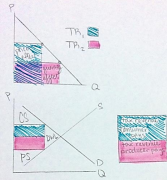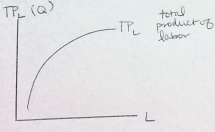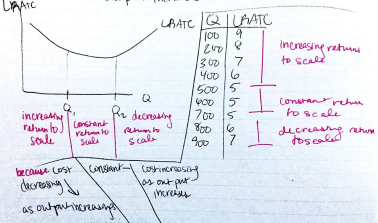×
Get Full Access to csulb - ECON 300 - Study Guide - Midterm
Get Full Access to csulb - ECON 300 - Study Guide - Midterm

×

CSULB / Economics / ECON 300 / Why is elasticity in demand always negative?

# Why is elasticity in demand always negative? Description

##### Description: Midterm 2 Study Guide: CH 5, CH 6
5 Pages 153 Views 5 Unlocks
Reviews

Econ 300 – Fundamentals of Economics

## Why is elasticity in demand always negative?Midterm 2 Study Guide

Ch 5 and 6 Outline

1. Chapter 5: Elasticity

a. Elasticity (e)

i. Measures the percent change in one variable when the other variable  changes on another variable; how responsive/elastic Y is to changes in X 1. �� =%Δ��

%Δ��

ii. Positive elasticity: percent change is in the same direction, meaning that  both X and Y will increase or decrease in change

b. Price Elasticity in Demand

i. Elasticity in demand is always negative.

1. Demand curve is a downward sloping curve. As X increases, Y

decreases.

2. �� =%Δ����

%Δ��

## How does budget share affect price elasticity for demand?3. %∆���� =��2−��1

2] %∆�� =��2−��1

[��2+��1 Don't forget about the age old question of What kind of waves are p-waves?

ii. After solving for e,

[��2+��1 2]

1. If |��| = 1, then unit is elastic

2. If |��| > 1, then demand is elastic or responsive to change and  %∆���� is greater than %∆��

3. If |��| < 1, then demand is not elastic or inelastic and %∆���� is less  than %∆��

## What does total revenue pertain to?4. Elasticity is undefined and perfectly elastic if %∆�� = 0

5. Elasticity is perfectly inelastic if �� = 0 or %∆���� = 0 If you want to learn more check out What does a fish's gastrovascular cavity consist of?

c. Factors that determine price elasticity for demand

i. Substitutes: demand becomes more elastic if close substitutes are readily  available.

ii. Budget share: the larger the share that an item in your budget accounts for,  the greater your incentive becomes to look for substitutes. Expensive  items tend to be more elastic to change.

iii. Time: demand is more elastic in the long run than the short run d. Cross Price Elasticity

i. Measures the price of one good and the quantity demanded for another  good

1. �� =%Δ����

%Δ����

2. If X and Y are substitutes, then �� > 0 and will be positive.

3. If X and Y are complements, then �� < 0 and will be negative. e. Income Elasticity of Demand

i. How sensitive demand is to change in incomeWe also discuss several other topics like Where do heterotrophs sources their food from?

ii. �� =%Δ��

%Δ��(I) = income

iii. If e is positive [e > 0], the good is a normal good.

iv. If e is negative [e < 0], the good is an inferior good

f. Elasticity and Total Revenue

i.

ii. Total Revenue (TR): total amount that sellers receive or the value of the  sales1. ���� = �� × ��

iii. Price effect: when price changes,

revenue changes

iv. Quantity effect: when quantity changes,

revenue changes

v. If Q effect is larger than P effect, then

TR increases.

vi. If Q effect is smaller than P effect, then

TR decreases.

g. Supply Elasticity

i. �� =%Δ����

%Δ��

h. Determining factors of supply elasticity

i. Whether inputs are unique and essential We also discuss several other topics like How does the mother behave in avoidant attachment style?

1. The easier it is to acquire the inputs, the higher the supply

elasticity will be.

i. The revenue from an excise tax

i. Excise tax: fixed amount of money paid per unit to the government 1. Governments use excise tax to provide incentive for people to  change behavior

2. Revenue from tax are paid by producers and consumers.

ii. Efficiency of tax

1. When tax is imposed, DWL occurs.

iii. Tax revenue isn’t always paid equally between consumers and producers.  The group with high elasticity will pay less because demand is less  elastic/responsive

j. Excise tax example:

i. The table shows 5 consumer WTP for 1 can of soda and 5 producer cost of  selling 1 can. An excise tax of \$0.40 is imposed. Without tax, what’s P*  and Q*? With tax, what’s P and Q?

Demand

Supply

Consumer

WTP

Producer

Cost

A

0.7

Z

0.1

B

0.6

Y

0.2

C

0.5

X

0.3

Don't forget about the age old question of Who is king ashoka?

D

0.4

W

0.4

E

0.3

V

0.5

1. Find out what consumers will buy and what producers are willing  to sell based on given values.

At price

Who’s willing to buy or sell

0.7

0.6

0.5

0.4

0.3

0.2

0.1

2. Find P* and Q* at equilibrium: at the price 0.4, 4 are willing to buy  and 4 are willing to sell because Consumers A-D are willing to buy  and Producers Z-W are willing to sell. Therefore, P* = 0.4 and Q*  = 4. If you want to learn more check out What do behaviorism and pragmatism have in common?

3. With tax at 0.4, subtract tax from possible prices.

At price with tax

Price before tax

Who’s willing to  buy or sell

0.3

0.7

0.2

0.6

0.1

0.5

The price with tax is 0.2 because Consumers B and C are willing to  pay (based on price before tax) and Producers Z and Y are willing  to sell. Therefore, P = 0.6 and Q = 2

4. Find CS and PS before and after the excise tax.

a. CS:

Price

WTP

CS

Total

CS

With tax

0.6

0.7

0.6

0.1

0

0.1

Without tax

0.4

0.7

0.6

0.5

0.4

0.3

0.2

0.1

0

0.6

CS lost due to tax is 0.5 because total CS with tax – total  CS without tax = amount of CS lost due to tax.

b. PS:

Price

Cost

PS

Total PS

With tax

0.6 (0.2 after  subtracting  tax)

0.2

0.1

0

0.1

0.1

Without

tax

0.4

0.4

0.3

0.2

0.1

0

0.1

0.2

0.3

0.6

PS lost due to tax is 0.5 because total PS with tax – total PS

without tax = amount of PS lost due to tax.

c. Government revenue is 0.8 because only 2 products are

sold when the tax is 0.4 per product.

ii. **if given a graph for given information, the tax is the price difference  between what consumer and producers pay.

2. Chapter 6: Production Function

a. Shows mathematical relationship between output and input; how output is  determined by input

i. �� = ��(��, ��) when k = capital L = labor Q = quantity of output 1. L and k are independent variables. Q is a dependent variable

ii. Fixed input: input that doesn’t change with the quantity of output

iii.iv. Variable input: input that changes

based on the quantity of output

v. Long run: period of time when all

inputs are varied

vi. Short run: period of time when at

least one input is fixed

b. Total Product Curve

i. Marginal product of an input: the

additional quantity of output that is

produced by using one more unit of that input

1. ���������������� �������������� ���� ���������� (������) =∆��

∆��

2. ���������������� �������������� ���� �������������� (������) =∆��

∆��

ii. Diminishing marginal product of input: firm has fixed input. After a  certain amount of labor, the less each worker can produce.

Productivity/efficiency decreases after a certain point.

iii. Diminishing marginal productivity: diminishing returns to an input (labor  or capital)

c. Costs

i. Fixed cost (FC): a cost that doesn’t depend on the quantity of output 1. If labor and quantity are 0 and total cost is listed, then the amount

listed for total cost is the fixed cost.

ii. Variable cost (VC): a cost that is dependent on the quantity of output iii. Marginal cost (MC): change in total cost with last unit of output;

additional cost incurred by producing one more unit

1. ���� =∆����

∆��TC = Total Cost Q = Quantity

iv. Average [total] cost (AC or ATC): cost per unit of output on average 1. ������ =������

2. ������ = ������ + ������

v. Average variable cost (AVC): variable cost per unit of output on average 1. ������ =������

vi. Average fixed cost (AFC): fixed cost per unit of output on average

1. ������ =������

d. Long Run vs Short Run

i. Total costs are higher in the short run than in the long run.

e. Returns to scale

i. There are increasing returns to scale (economies of scale) when long run  ATC declines as output increases

ii. There are decreasing returns to scale (diseconomies of scale) when short run ATC increases as output increases.

iii. There are constant returns to scale when short run ATC is constant as output increases.f. Summary of formulas

VC

TC

AVC

AFC

ATC

MC

MPL

TC - FC

FC+VC

����

��

����

��

AVC+AFC or

����

��

∆����

∆��

∆��

∆��

Page ExpiredIt looks like your free minutes have expired! Lucky for you we have all the content you need, just sign up here